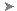# WMFIN (Command)

Imports a Windows metafile.

Find

WMF files can contain both vector and raster information, however only the vector information is imported. WMF files are inserted as a block reference and can be resized and printed without losing resolution.

The Import WMF dialog box (a standard file selection dialog box) is displayed.

In the Import WMF dialog box, if you click ToolsOptions, the WMF In Options dialog box is displayed. You can also open this dialog box directly by using WMFOPTS.

The following prompts are displayed.

### Insertion Point

Places a copy of the metafile with its base point at the specified insertion point.

X Scale Factor

Sets the X and Y scale factors.

All X and Y dimensions of the metafile are multiplied by the X and Y scale factors supplied. The metafile is rotated by the angle specified, using the insertion point as the center of rotation.

Corner

Defines the X and Y scales at the same time, using the insertion point and another point as the corners of a box. The X and Y dimensions of the box become the X and Y scale factors. The insertion point is the first corner.

The rotation angle sets the angle of the WMF file.

XYZ

Scales the metafile in all three dimensions.

If you enter c, you specify a corner point. The specified point and the insertion point determine the X and Y scale factors for the WMF file.

The rotation angle sets the angle of the WMF file.

### Scale

Sets the scale factor for the X, Y, and Z axes. The scale for the Z axis is the absolute value of the specified scale factor.

### X

Sets the X scale factor.

### Y

Sets the Y scale factor.

### Z

Sets the Z scale factor.

### Rotate

Sets the angle of insertion for the WMF file.

• X Scale Factor
• Corner
• XYZ

### PScale

Sets the temporary scale factor for the X, Y, and Z axes to control the display of the WMF file as it is dragged into position.

• X Scale Factor
• Corner
• XYZ

### PX

Sets the temporary scale factor for the X axis to control the display of the WMF file as it is dragged into position.

• X Scale Factor
• Corner
• XYZ

### PY

Sets the temporary scale factor for the Y axis to control the display of the WMF file as it is dragged into position.

• X Scale Factor
• Corner
• XYZ

### PZ

Sets the temporary scale factor for the Z axis to control the display of the WMF file as it is dragged into position.

• X Scale Factor
• Corner
• XYZ

### PRotate

Sets the temporary rotation angle of the WMF file as it is dragged into position.

• X Scale Factor
• Corner
• XYZ